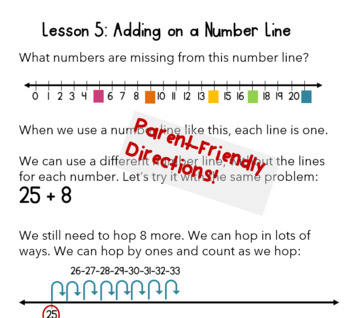1st - 2nd
Subjects
Standards
Resource Type
Formats Included
• PDF
Pages
126 pages

### Description

Boost your First Grader's math skills and build number concepts with our 6-week skill building packet!

This adorable and engaging set of of parent-friendly resources blends hands-on activities, games, and practice pages to keep kids learning all summer long!

This complete curriculum address all the CCSS-M standards for 1st Grade, including:

• Addition and subtraction models to 20
• True and false number sentences
• Fact families and number bonds
• Place value to 100
• Two-digit numbers
• Multiples of ten, ten more, ten less
• Fluently adding and subtracting within 10
• Comparing numbers and length
• Telling time to the hour and half-hour

We have summer packets for Grades K-4, with Grade 5 coming out soon! Check out our TpT store for all our curriculum!

Total Pages
126 pages
N/A
Teaching Duration
N/A
Report this Resource to TpT
Reported resources will be reviewed by our team. Report this resource to let us know if this resource violates TpT’s content guidelines.

### Standards

to see state-specific standards (only available in the US).
Use addition and subtraction within 20 to solve word problems involving situations of adding to, taking from, putting together, taking apart, and comparing, with unknowns in all positions, e.g., by using objects, drawings, and equations with a symbol for the unknown number to represent the problem.
Solve word problems that call for addition of three whole numbers whose sum is less than or equal to 20, e.g., by using objects, drawings, and equations with a symbol for the unknown number to represent the problem.
Apply properties of operations as strategies to add and subtract. If 8 + 3 = 11 is known, then 3 + 8 = 11 is also known. (Commutative property of addition.) To add 2 + 6 + 4, the second two numbers can be added to make a ten, so 2 + 6 + 4 = 2 + 10 = 12. (Associative property of addition.)
Understand subtraction as an unknown-addend problem. For example, subtract 10 – 8 by finding the number that makes 10 when added to 8.
Relate counting to addition and subtraction (e.g., by counting on 2 to add 2).